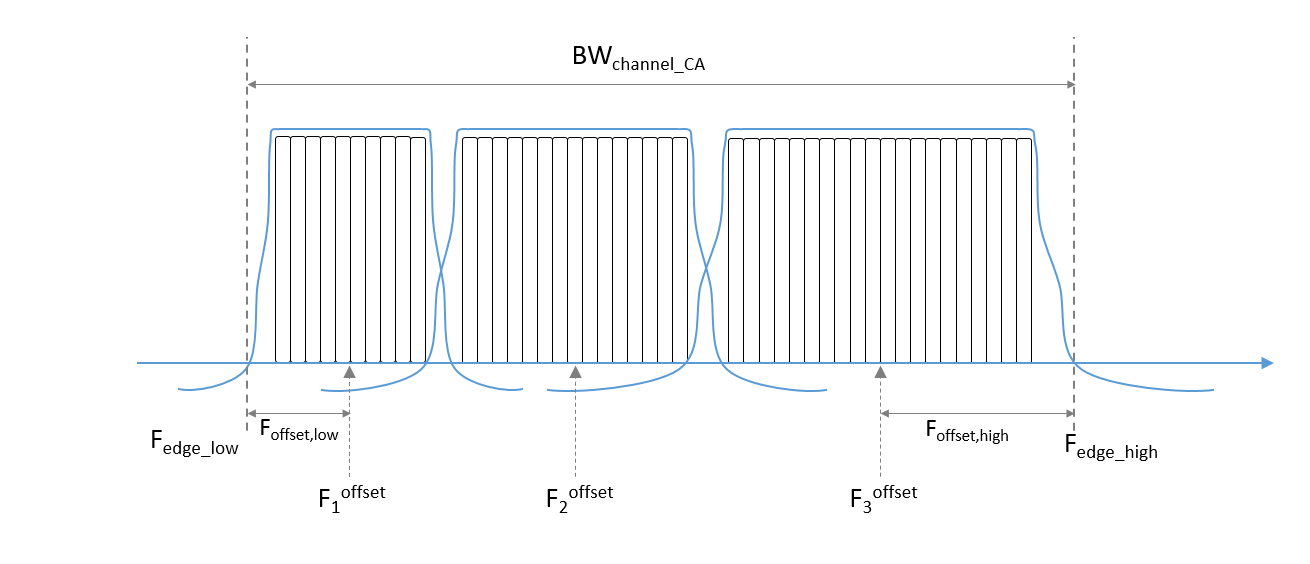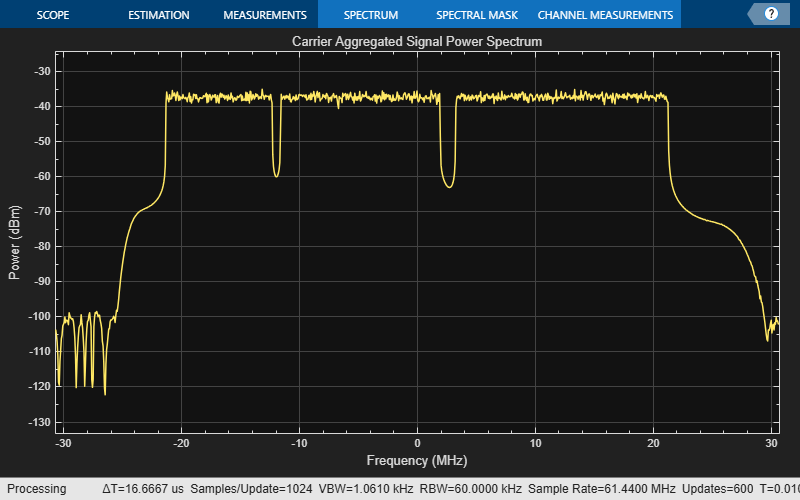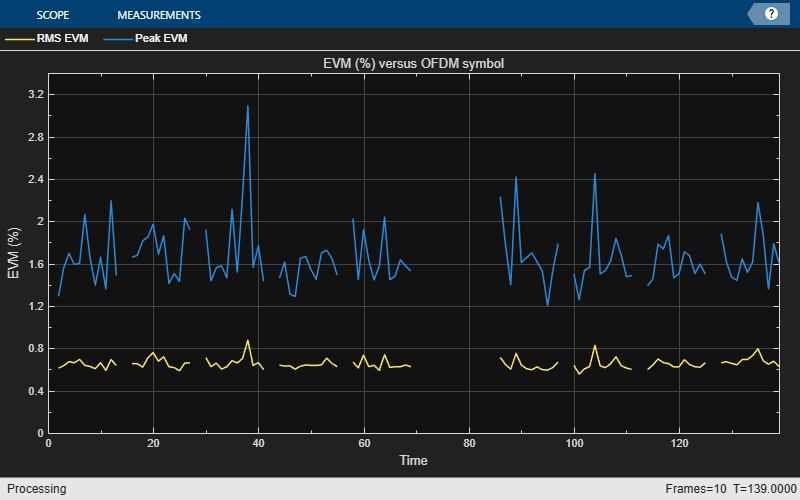## Release 12 Downlink Carrier Aggregation Waveform Generation, Demodulation and Analysis

This example shows how multiple downlink carriers can be generated, aggregated and further demodulated using the LTE Toolbox™.

### Introduction

This example models an LTE Release 12 waveform with Carrier Aggregation (CA). The number of subcarriers and their bandwidth can be specified as a parameter. An intra-band contiguous CA case is considered.

To generate an aggregated downlink waveform an eNodeB is configured for each component carrier. The component carrier parameters are calculated and used to generate a modulated waveform for each eNodeB configuration. All the CC modulated waveforms are resampled to a common sampling rate so they can be combined to create an aggregated waveform.

### Number of Component Carriers and Bandwidths

A vector `NDLRB` specifies the number of resource blocks (RBs) for each component carrier (CC). The length of this vector corresponds to the number of CCs. The elements of `NDLRB` must be in the set {6, 15, 25, 50, 75, 100} RBs. TS 36.101 Table 5.6A.1-1 [ 1 ] lists the valid combinations of bandwidths for carrier aggregation.

```% Configure the set of NDLRB values to describe the carriers to be % aggregated NDLRB = [50 75 100]; % Establish the number of component carriers numCC = numel(NDLRB); if numCC<2 error('Please specify more than one CC bandwidth value.'); end ```

### Component Carrier Configuration

A configuration structure is generated for each CC using lteRMCDL. The configuration structures for all CCs are stored in a cell array.

```% Configure the number of subframes to generate numSubframes = 10; % CC configuration enb = cell(1,numCC); for i = 1:numCC switch NDLRB(i) case 6 enb{i} = lteRMCDL('R.4'); case 15 enb{i} = lteRMCDL('R.5'); case 25 enb{i} = lteRMCDL('R.6'); case 50 enb{i} = lteRMCDL('R.7'); case 75 enb{i} = lteRMCDL('R.8'); case 100 enb{i} = lteRMCDL('R.9'); otherwise fprintf('Not a valid number of resource blocks: %d\n',... NDLRB(i)); return end enb{i}.NDLRB = NDLRB(i); enb{i}.Bandwidth = hNRBToBandwidth(NDLRB(i)); enb{i}.TotSubframes = numSubframes; enb{i}.PDSCH.PRBSet = (0:enb{i}.NDLRB-1).'; enb{i}.PDSCH.RVSeq = 0; enb{i}.NCellID = 10; end ```

### Channel Estimator Configuration

The channel estimator at the receiver end is parameterized using the structure `cec` defined below.

```cec = struct; % Channel estimation config structure cec.PilotAverage = 'UserDefined'; % Type of pilot symbol averaging cec.FreqWindow = 15; % Frequency window size cec.TimeWindow = 15; % Time window size cec.InterpType = 'Cubic'; % 2D interpolation type cec.InterpWindow = 'Centered'; % Interpolation window type cec.InterpWinSize = 1; % Interpolation window size ```

### Carrier Aggregation Parameter Calculation

To perform carrier aggregation the frequency parameters described in TS 36.101, Sections 5.6 and 5.7 [ 1 ] are calculated. These parameters are summarised in the following figure.This results in three variables:

• `F_c` is a vector containing the center frequency of each CC

• `ccSpacing` contains the spacing between CC in MHz

• `BW_channel_CA` is the aggregated channel bandwidth of all CCs

In the code below we first calculate the value for all the CCs assuming the lower one is centered at baseband (0 Hz). Once the `BW_channel_CA` is calculated all the values are shifted so the center of the aggregated bandwidth is located at baseband (0 Hz).

```% Define Delta_f1 parameter in MHz for nominal guard band and frequency % offset calculations. In the downlink Delta_f1 is the subcarrier spacing % (TS 36.101, Table 5.6A-1) Delta_f1 = 0.015; % in MHz, LTE subcarrier spacing in MHz maxBW = hNRBToBandwidth(max(NDLRB)); F_c = zeros(1,numCC); ccSpacing = zeros(1,numCC-1); % CC spacing % Calculate nominal guard band, TS 36.101 5.6A-1 nominalGuardBand = 0.05*maxBW-0.5*Delta_f1; % Initially assume lower carrier frequency is at baseband F_c(1) = 0; % Calculate CC spacing and carrier values for k = 2:numCC ccSpacing(k-1) = hCarrierAggregationChannelSpacing( ... enb{k-1}.Bandwidth, enb{k}.Bandwidth); F_c(k) = F_c(k-1) + ccSpacing(k-1); end % Calculate lower and higher frequency offsets, TS 36.101 5.6A F_offset_low = (0.18*NDLRB(1)+Delta_f1)/2 + nominalGuardBand; F_offset_high = (0.18*NDLRB(end)+Delta_f1)/2 + nominalGuardBand; % Calculate lower and higher frequency edges, TS 36.101 5.6A F_edge_low = F_c(1) - F_offset_low; F_edge_high = F_c(end) + F_offset_high; % Calculate aggregated channel bandwidth, TS 36.101 5.6A BW_channel_CA = F_edge_high - F_edge_low; fprintf('BW_channel_CA: %0.4f MHz\n',BW_channel_CA); % Calculate shift to center baseband shiftToCenter = -1*(BW_channel_CA/2 + F_edge_low); % Aggregated bandwidth centered at baseband F_c = F_c + shiftToCenter; F_edge_low = F_c(1) - F_offset_low; F_edge_high = F_c(end) + F_offset_high; % Display frequency band edges fprintf('F_edge_low: %0.4f MHz\n',F_edge_low); fprintf('F_edge_high: %0.4f MHz\n',F_edge_high); fprintf('F_offset_low: %0.4f MHz\n',F_offset_low); fprintf('F_offset_high: %0.4f MHz\n',F_offset_high); % Display carrier frequencies fprintf('\n'); for i = 1:numCC fprintf('Component Carrier %d:\n',i); fprintf(' Fc: %0.4f MHz\n', F_c(i)); end ```
```BW_channel_CA: 44.6000 MHz F_edge_low: -22.3000 MHz F_edge_high: 22.3000 MHz F_offset_low: 5.5000 MHz F_offset_high: 10.0000 MHz Component Carrier 1: Fc: -16.8000 MHz Component Carrier 2: Fc: -4.8000 MHz Component Carrier 3: Fc: 12.3000 MHz ```

### Required Oversampling Rate Calculation

The required oversampling factors for each component carrier `OSRs` are calculated for a common sampling rate for the aggregated signal.

```% Bandwidth utilization of 85% bwfraction = 0.85; % Calculate sampling rates of the component carriers CCSR = zeros(1,numCC); for i = 1:numCC info = lteOFDMInfo(enb{i}); CCSR(i) = info.SamplingRate; end % Calculate overall sampling rate for the aggregated signal % Calculate the oversampling ratio (for the largest BW CC) to make sure the % signal occupies 85% (bwfraction) of the total bandwidth OSR = (BW_channel_CA/bwfraction)/(max(CCSR)/1e6); % To simplify the resampling operation choose an oversampling ratio which % is a power of 2: calculate the next power of two above OSR OSR = 2^ceil(log2(OSR)); SR = OSR*max(CCSR); fprintf('\nOutput sample rate: %0.4f Ms/s\n\n',SR/1e6); % Calculate individual oversampling factors for the component carriers OSRs = SR./CCSR; ```
```Output sample rate: 61.4400 Ms/s ```

### Waveform Generation and Carrier Aggregation

lteRMCDLTool is used to generate the waveform for each CC. Each of them is resampled to a common sampling rate, frequency modulated to the appropriate center frequency and finally added together to form the aggregated signal.

```% Generate component carriers tx = cell(1,numCC); for i = 1:numCC tx{i} = lteRMCDLTool(enb{i},randi([0 1],1000,1)); tx{i} = resample(tx{i},OSRs(i),1)/OSRs(i); tx{i} = hCarrierAggregationModulate(tx{i},SR,F_c(i)*1e6); end % Superpose the component carriers waveform = tx{1}; for i = 2:numCC waveform = waveform + tx{i}; end ```

### Plot Carrier Aggregation Waveform Spectrum

The power spectrum of the carrier aggregated signal is displayed using hCarrierAggregationPlotSpectrum.m. In the spectrum the individual carrier bandwidths are visible. Note that the center of the aggregated bandwidth is located at baseband (0 Hz), i.e. in this example the signal is not modulated to RF.

```specPlot = hCarrierAggregationPlotSpectrum(waveform,SR,... 'Power Spectrum of Carrier Aggregation',{'Signal spectrum'}); ```### Demodulation and Filtering of CC of Interest

This section demodulates, filters and downsamples one of the CCs. The steps followed are:

• Demodulate the CC of interest and bring it to baseband (0 Hz).

• Filter out neighboring CCs and downsample. A suitable filter is designed to remove the unwanted neighboring CCs in the downsampling process. The filter design will have an impact on the quality and EVM of the recovered signal. Filters may need to be tweaked for different values of bandwidth and CC to demodulate.

We start by selecting the CC to demodulate and designing the appropriate downsampling filter. Passband and stopband frequencies are calculated.

```% Select CC to demodulate CCofInterest = 1; if CCofInterest > numCC || CCofInterest <= 0 error('Cannot demodulate CC number %d, there are %d CCs\n',... CCofInterest, numCC) ; end % Define downsampling filter order filterOrder = 201; % Precalculate passband and stopband values for all CC firPassbandVec = (0.18*NDLRB+Delta_f1)/2 / (SR/1e6/2); firStopbandVec = hCarrierAggregationStopband(ccSpacing,NDLRB,SR); % Define passband and stopband for carrier of interest firPassband = firPassbandVec(CCofInterest); firStopband = firStopbandVec(CCofInterest); % Extract and decode CC of interest fprintf(1,'Extracting CC number %d: \n', CCofInterest); % Pad signal with zeros to take into account filter transient response % length waveform = [waveform; zeros(filterOrder+1,size(waveform,2))]; % Demodulate carrier of interest demodulatedCC = ... hCarrierAggregationModulate(waveform,SR,-F_c(CCofInterest)*1e6); % Downsampling is done in two stages if the filter is too narrow. This % eases the filter design requirements. If this is the case an initial % downsampling factor of 4 is applied. You may want to consider a different % filter design in this initial stage if the quality of the resulting % signal is not acceptable. if (firStopband < 0.1) % Down-sample by 4 SRC = 4; demodulatedCC = resample(demodulatedCC,1,SRC); % Update pass and stopband to take first downsampling into account firPassband = firPassband * SRC; firStopband = firStopband * SRC; else % No pre-filter SRC = 1; end % Design lowpass filter to filter out CC of interest frEdges = [0 firPassband firStopband 1]; firFilter = dsp.FIRFilter; firFilter.Numerator = firpm(filterOrder,frEdges,[1 1 0 0]); ```
```Extracting CC number 1: ```

The response of the designed filter is displayed.

```fvtool(firFilter,'Analysis','freq'); ```The demodulated waveform is then filtered to extract the CC of interest. The spectrum of the demodulated waveform before and after the filtering is plotted.

```% Filter the signal to extract the component of interest rxWaveform = firFilter(demodulatedCC); % Plot the demodulated and filtered signal spectra filteredSpecPlot = ... hCarrierAggregationPlotSpectrum([demodulatedCC, rxWaveform],SR,... 'Demodulated and Filtered Waveform Power Spectrum',... {'Carrier aggregated signal' 'Filtered signal'}); ```At this point the filtered waveform can be downsampled to its baseband rate.

```rxWaveform = downsample(rxWaveform,OSRs(CCofInterest)/SRC); ```

### Synchronization

Synchronization is applied to the resulting signal.

```% Parameters for CC of interest eNodeB = enb{CCofInterest}; PDSCH = eNodeB.PDSCH; % Synchronize received waveform offset = lteDLFrameOffset(eNodeB, rxWaveform); rxWaveform = rxWaveform(1+offset:end, :); ```

### EVM Measurements and PDSCH Decoding

The code below provides per subframe and average EVM measurements. Plots with the EVM versus time, resource block and subcarriers are also displayed.

The PDSCH of the recovered signal is decoded and the resulting CRC is checked for errors.

```% channel estimation structure for EVM measurement cecEVM = cec; cecEVM.PilotAverage = 'TestEVM'; [evmmeas, plots] = hPDSCHEVM(enb{CCofInterest},cecEVM,rxWaveform); % OFDM demodulation rxGrid = lteOFDMDemodulate(eNodeB,rxWaveform); % Get the number of received subframes and OFDM symbols per subframe dims = lteOFDMInfo(eNodeB); samplesPerSubframe = dims.SamplingRate/1000; nRxSubframes = floor(size(rxWaveform, 1)/samplesPerSubframe); eNodeB.TotSubframes = 1; resGridSize = lteResourceGridSize(eNodeB); L = resGridSize(2); disp('Decode transmitted subframes and check CRC.'); for n=0:nRxSubframes-1 % extract subframe rxSubframe = rxGrid(:,(1:L)+(n*L),:); % transport block size for current subframe eNodeB.NSubframe = n; trBlkSize = PDSCH.TrBlkSizes(n+1); % Channel estimation [estChannelGrid, noiseEst] = lteDLChannelEstimate( ... eNodeB, cec, rxSubframe); % Perform deprecoding, layer demapping, demodulation and descrambling % on the received data using the channel estimate [rxEncodedBits, pdschsymbols] = ltePDSCHDecode(eNodeB,PDSCH, ... rxSubframe*(10^(-PDSCH.Rho/20)),estChannelGrid,noiseEst); % Decode DownLink Shared Channel (DL-SCH) [decbits,crc] = ... lteDLSCHDecode(eNodeB,PDSCH,trBlkSize,rxEncodedBits{1}); if crc disp(['Subframe ' num2str(n) ': CRC failed']); else disp(['Subframe ' num2str(n) ': CRC passed']); end end ```
```Low edge EVM, subframe 0: 0.647% High edge EVM, subframe 0: 0.629% Low edge EVM, subframe 1: 0.681% High edge EVM, subframe 1: 0.649% Low edge EVM, subframe 2: 0.680% High edge EVM, subframe 2: 0.676% Low edge EVM, subframe 3: 0.675% High edge EVM, subframe 3: 0.641% Low edge EVM, subframe 4: 0.639% High edge EVM, subframe 4: 0.647% Low edge EVM, subframe 6: 0.698% High edge EVM, subframe 6: 0.642% Low edge EVM, subframe 7: 0.645% High edge EVM, subframe 7: 0.648% Low edge EVM, subframe 8: 0.700% High edge EVM, subframe 8: 0.647% Low edge EVM, subframe 9: 0.694% High edge EVM, subframe 9: 0.684% Averaged low edge EVM, frame 0: 0.674% Averaged high edge EVM, frame 0: 0.652% Averaged EVM frame 0: 0.674% Averaged overall EVM: 0.674% Decode transmitted subframes and check CRC. Subframe 0: CRC passed Subframe 1: CRC passed Subframe 2: CRC passed Subframe 3: CRC passed Subframe 4: CRC passed Subframe 5: CRC passed Subframe 6: CRC passed Subframe 7: CRC passed Subframe 8: CRC passed Subframe 9: CRC passed ```### Appendix

This example uses the following helper functions:

### Selected Bibliography

1. 3GPP TS 36.101 "User Equipment (UE) radio transmission and reception"